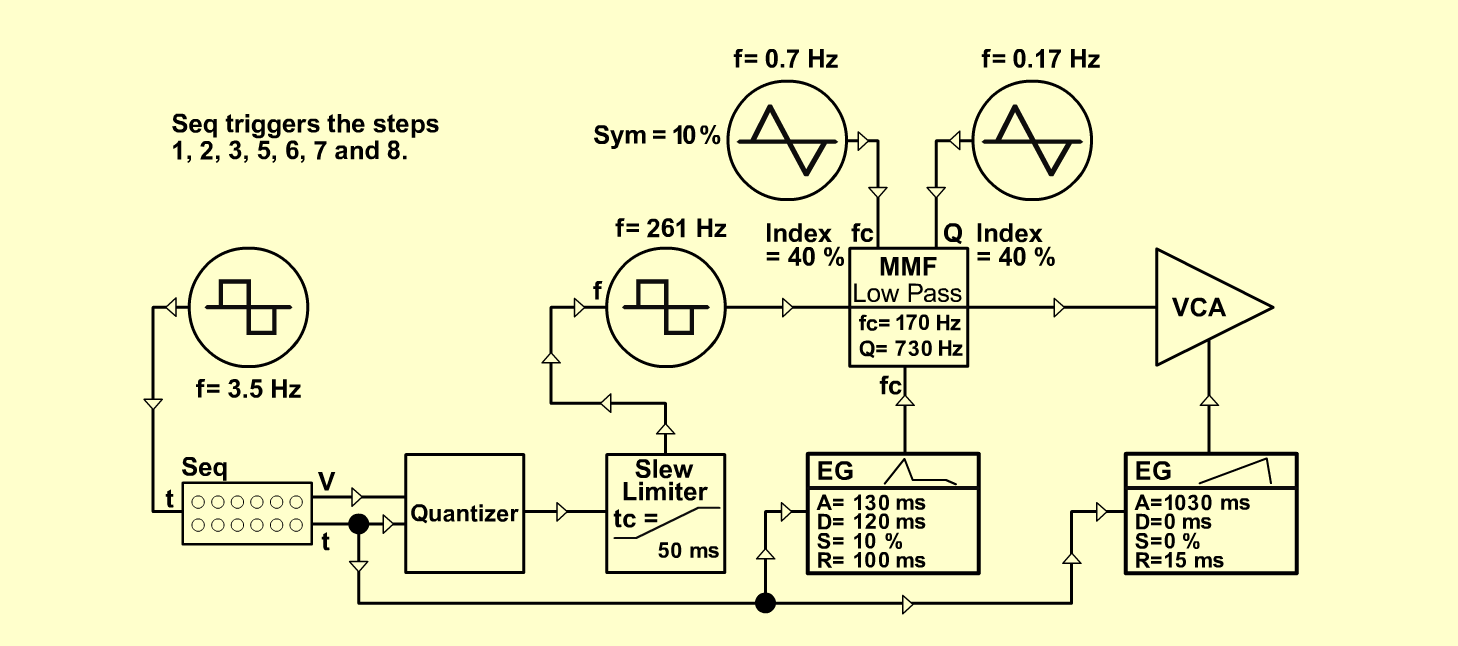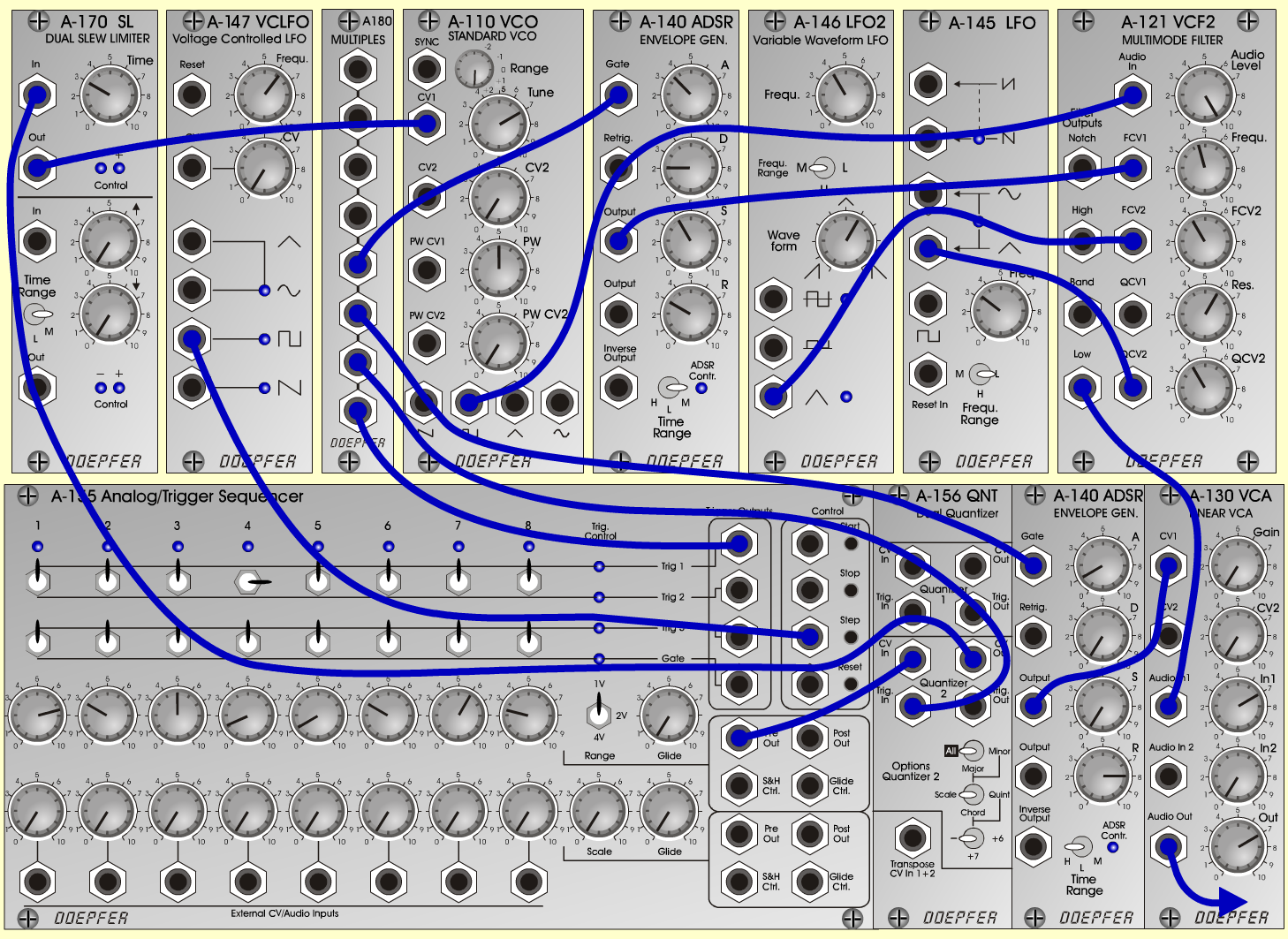Fi's SequenceTextureD o e p f e r A - 100 Connections: Settings: A-147 (Square)  <=>  A-155 (Step/Clock) A-155 (Trig 1)  <=>  A-180 A-180  <=>  A-156 (Trig In/Q2) A-155 (Pre Out/1)  <=>  A-156 (CV In/Q2) A-156 (CV Out/Q2)  <=>  A-170 (In) A-170 (Out)  <=>  A-110 (CV 1) A-110 (Square)  <=>  A-121 (Audio In) A-180  <=>  A-140/1 (Gate) A-140/1 (Output)  <=>  A-121 (FCV 1) A-146 (Triangle)  <=>  A-121 (FCV 2) A-145 (Triangle)  <=>  A-121 (QCV 2) A-121 (Low)  <=>  A-130 (Audio In) A-180  <=>  A-140/2 (Gate) A-140/2 (Output)  <=>  A-130 (CV1) A-130 (Audio Out)  <=> Amplifier A-110 (Range = +3, Tune = 7, PW = 5) A-121 (Audio Level = 10, Freq = 4.5, FCV 2 = 4, Res = 6, QCV 2 = 4) A-130 (Gain = 0, Audio In 1 = 7,  Audio Out = 7) A-145 (Frq = 3.25, Range = L) A-146 (Freq = 4, Wave Form = 6, Range = M) A-147 (Freq = 6.25) A-140/1 (A = 3.5, D = 2, S = 4, R = 3, Range = M) A-140 /2(A = 1, D = 0, S = 0, R = 8, Range = H) A-155 (Row Up: 1 = 7.5, 2 = 3, 3 = 5, 4 = 1.2, 5 = 1, 6 = 3, 7 = 6, 8 = 2.5, Range = 1V, Glide = 0, Trig 1: 1 = up, 2 = up, 3 = up, 4 = mid, 5 = up, 6 = up, 7 = up, 8 = up) A-156 (Options Q2: 1 = All, 2 = Scale, 3 = -) A-170 (Time = 3) David Salter, September 2001Sound sample Fi's Sequence
br>